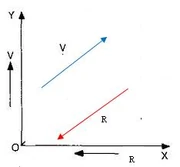## FANDOM

225 Pagesindirectly proportional relationships

## About

In mathematics, indirectly proportional constants are two quantities that are NOT constant and in the sense of the word NOT moving in the same direction of each other. For example, as one positive number increases, then the other number decreases at the same negative rate too. The analogy works for negative numbers too as long as the numbers are moving negatively and in the opposite positive direction.

In engineering, we use the three fundamental electrical quantities: current, voltage, and resistance to calculate ohms law. We have used Ohm's law to state that the electrical current (I) flowing in an circuit is indirectly [inversely] proportional to the resistance (R).

Why? Because increasing the resistance of the circuit will lower the current flow if the voltage is not changed.